# Java Program to Add Two Matrix Using Multi-dimensional Arrays

In this tutorial you will learn about the Java Program to Add Two Matrix Using Multi-dimensional Arrays and its application with practical example.

## Java Program to Add Two Matrices Using Multi-dimensional Arrays

In this tutorial, we will learn to create a Java program that will Add Two Matrix Using Multi-dimensional Arrays using Java programming.

## Prerequisites:-

Before starting with the tutorial, we assume that you are the best aware of the following Java programming topics:

• Operators in Java Programming.
• Basic Input and Output function in Java Programming.
• Basic Java programming.
• For loop in Java programming.

## Program to Add Two Matrices Using Multi-dimensional Arrays

In this program, First, we will take two matrices in input from the user using looping statements for the program. Then secondly, we will add the two matrices using the for loops into a third matrix. At last, we will print the added matrix to the user using the system. Out method.

Algorithm:-

With the help of this program, we can Add Two Matrices Using Multi-dimensional Arrays

## Output:-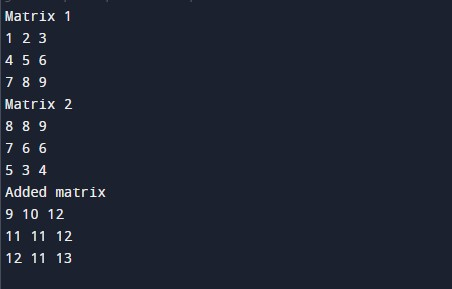In the above program, we have first initialized the required variable.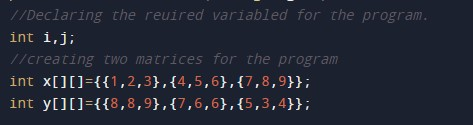• x[][] = it will hold the integer value.
• y[][] = it will hold the integer value.
• sum[][] = it will hold the integer value.
• i= it will hold the integer value.
• j= it will hold the integer value.

Printing the first matrix to the user.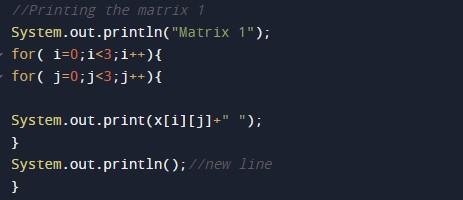Printing the second matrix to the user.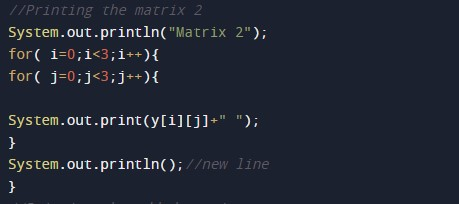Adding the two matrices into 1 and printing the added matrix to the user.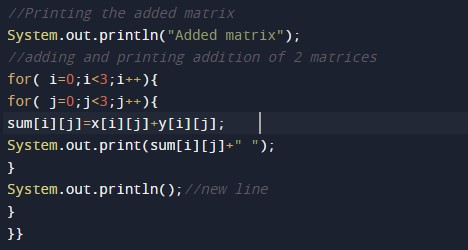In this tutorial we have learn about the Java Program to Add Two Matrix Using Multi-dimensional Arrays and its application with practical example. I hope you will like this tutorial.